# Samacheer Kalvi 12th Maths Solutions Chapter 7 Applications of Differential Calculus Ex 7.6

## Tamilnadu Samacheer Kalvi 12th Maths Solutions Chapter 7 Applications of Differential Calculus Ex 7.6

Question 1.
Find the absolute extrema of the following functions on the given closed interval.
(i) f(x) = x3 – 12x + 10; [1, 2]
(ii) f(x) = 3x4 – 4x3 ; [-1, 2]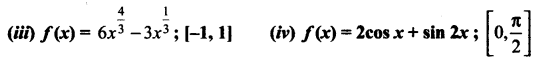Solution: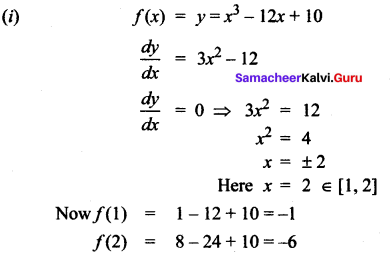∴ Absolute maximum is – 1 and absolute minimum is – 6

(ii) f(x) = 3x4 – 4x3
f'(x) = 12x3 – 12x2
f'(x) = 0 ⇒ 12x2(x – 1) = 0
⇒ x = 0 or x = 1
[Here x = 0, 1 ∈ [-1, 2]]
Now f (-1) = 4
f(0) = 0
f(1) = -1
f(2) = 16
so absolute maximum = 16 and absolute minimum = -1(iii)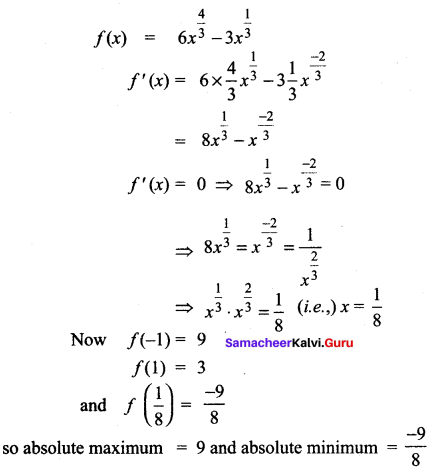(iv) f(x) = 2 cos x + sin 2x
f'(x) = -2 sin x + 2 cos 2x
f'(x) = 0 ⇒ cos 2x = sin x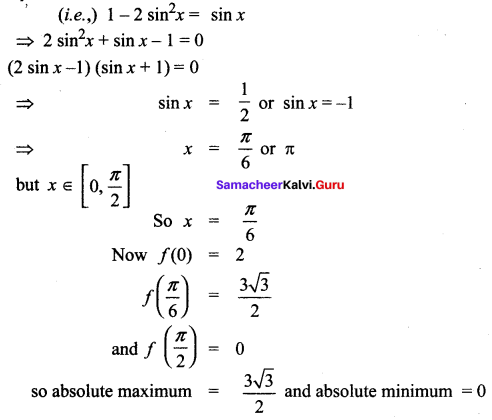Question 2.
Find the intervals of monotonicities and hence find the local extremum for the following functions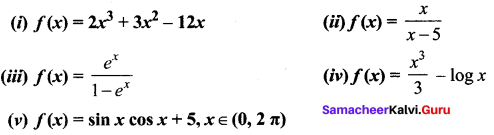Solution:
(i) f(x) = 2x3 + 3x2 – 12x
f'(x) = 6x2 + 6x – 12
f'(x) = 0 ⇒ 6(x2 + x – 2) = 0
(i.e.,) 6(x + 2)(x – 1) = 0
⇒ x = -2 or 1
Taking the points in the number line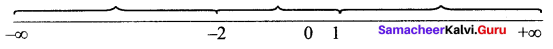The intervals are (-∞, -2), (-2, 1), (1, ∞)
when x ∈ (-∞, -2), f'(x) = 6 (-1) (-4) = +ve
say x = – 3
⇒ f(x) is strictly increasing in the interval (-∞, -2) when x ∈ (-2, 1), f’ (x) = 6 (2) (-1) = -ve
say x = 0
⇒ f(x) is strictly decreasing in the interval (-2, 1)
when x ∈ (1, ∞), f'(x) = 6 (4) (+1) = + ve say x = 2
⇒ f(x) is strictly increasing in (1, ∞)
Since f(x) changes from +ve to – ve when passing through -2, the first derivative, test tells us there is a local maximum at x = -2 and the local maximum value is f(-2) = 20.
Again f'(x) changes from – ve to +ve when passing through 1 ⇒ there is a local minimum at x = 1 and the local minimum value is f( 1) = -7. So (1 )f(x) is strictly increasing on (-∞, -2) and (1, ∞). And (2)f(x) is strictly decreasing on (-2, 1)
The local maximum = 20 and the local minimum = -7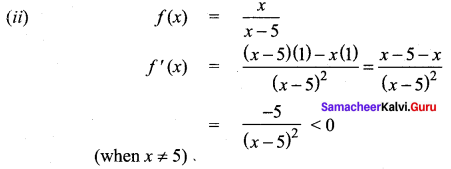f(x) is strictly decreasing on (-∞, 5) and (5, ∞)
And there is no local extremum
(iii)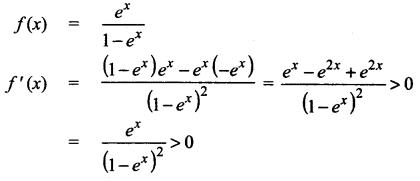For all x values, so f(x) is strictly increasing in (-∞, ∞) and there is no local extremum.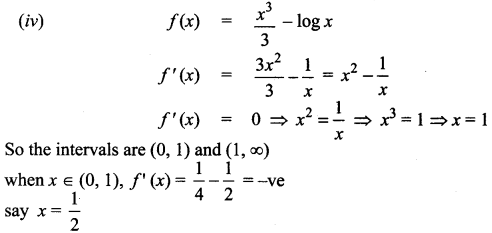⇒ f(x) is strictly decreasing in (0, 1)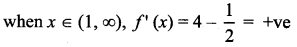say x = 2
⇒ f(x) is strictly increasing in (1, ∞)
since f'(x) changes from -ve to +ve at x = 1, there is a local minimum at x = 1 and the local minimum values is f(1) = $$\frac{1}{3}-0=\frac{1}{3}$$
So the function is strictly decreasing on (0, 1) and strictly increasing on (1, ∞) and the local minimum value is $$\frac{1}{3}$$
(v)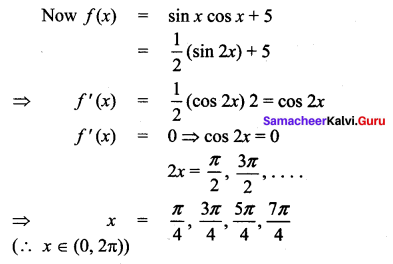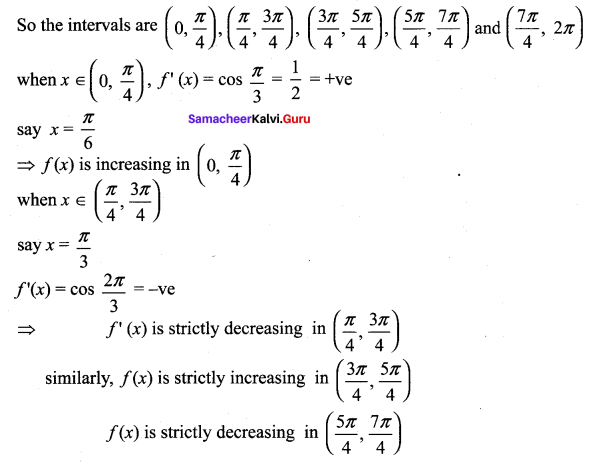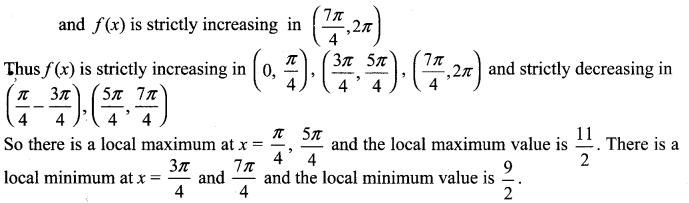### Samacheer Kalvi 12th Maths Solutions Chapter 7 Applications of Differential Calculus Ex 7.6 Additional Problems

Find the absolute maximum and absolute minimum values of f on the given interval.

Question 1.
f(x) = 1 – 2x – x2, [-4, 1]
Solution:
f(x) = 1 – 2x – x2
f'(x) = -2 – 2x
f'(x) = 0 ⇒ 2x = -2 ⇒ x = -1; The points are -4, -1, 1
At x = -4, f(x) = 1 – 2(-4) – (-4)2 = 1 + 8 – 16 = -7
At x = -1, f(x) = 1 – 2 (-1) – (-1)2 = 1 + 2 – 1 = 2
At x = 1, f(x) = 1 – 2 (1) – (1)2 = 1 – 2 – 1 = – 2
Therefore, the absolute minimum is -7 and the absolute maximum is 2.

Question 2.
f(x) = x3 – 12x + 1, [-3, 5]
Solution:
f(x) = x3 – 12x + 1
f'(x) = 3x2 – 12
f'(x) = 0 ⇒ 3x2 – 12 = 0
3x2 = 12 ⇒ x2 = 4 ⇒ x = ±2
The x values are -3, -2, 2, 5
f(x) (at x = -3) = (-3)3 – 12 (-3) + 1 = -27 + 36 + 1 = 10
f(x) (at x = -2) = (-2)3 – (12)(-2) + 1 = -8 + 24 + 1 = 17
f(x) (at x = 2) = 23 – 12 (2) + 1 = 8 – 24 + 1 = -15
f(x) (at x = 5) = 53 – 12 (5) + 1 = 125 – 60 + 1 = 66
From the above four values 10,17,-15 and 66, we see that absolute maximum is 66 and the absolute minimum is -15.

Question 3.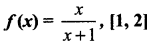Solution: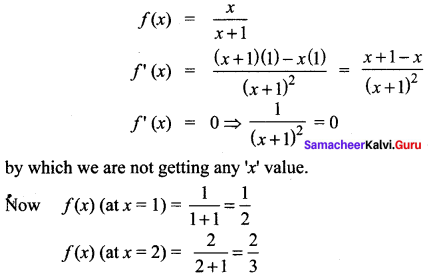So, the absolute maximum is $$\frac{2}{3}$$ and the absolute minimum is $$\frac{1}{2}$$Question 4.
f(x) = sin x + cos x, [0, π/3]
f'(x) = sin x + cos x
f'(x) = cos x – sin x
f’ (x) = 0 ⇒ cos x – sin x = 0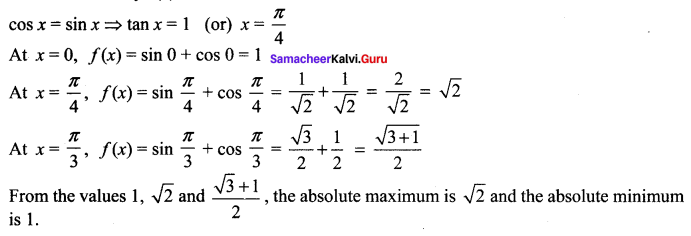Question 5.
f(x) = x – 2 cos x, [-π, π]
Solution:
f(x) = x – 2 cos x
f'(x) = 1 + 2 sin x
f'(x) = 0 ⇒ 1 + 2 sin x = 0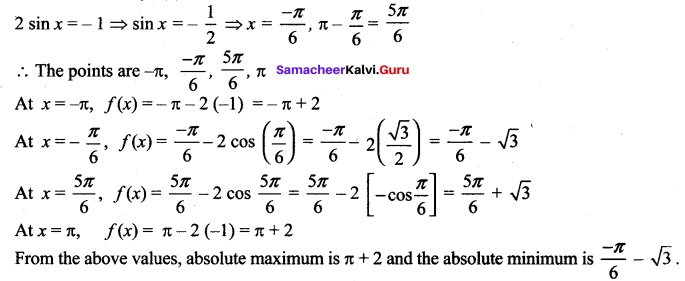From the above values, absolute maximum is π + 2 and the absolute minimum is $$\frac{-\pi}{6}-\sqrt{3}$$

From the local maximum and minimum values of the following functions.

Question 6.
2x3 + 5 x2 – 4x
Solution: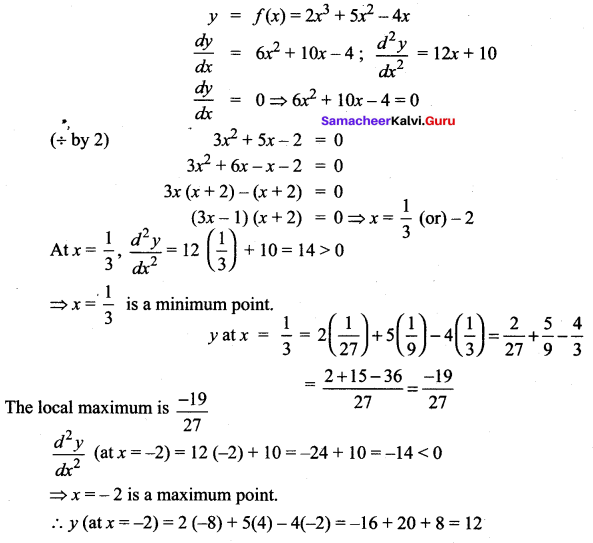Local maximum = 12Question 7.
t + cos t
Solution: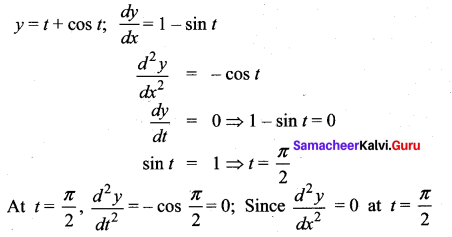t = $$\frac{\pi}{2}$$ is neither a maximum point nor a minimum point. So there is no maximum or minimum.